Which is a wrong statement :

(1) The Wheatstone bridge is most sensitive when all the four resistances are of the same order

(2) In a balanced Wheatstone bridge, interchanging the positions of galvanometer and cell affects the balance of the bridge

(3) Kirchhoff's first law (for currents meeting at a junction in an electric circuit) expresses the conservation of charge

(4) The rheostat can be used as a potential divider

Concept Questions :-

Wheatstone bridge
High Yielding Test Series + Question Bank - NEET 2020

Difficulty Level:

AB is a wire of uniform resistance. The galvanometer G shows no current when the length AC = 20cm and CB = 80 cm. The resistance R is equal to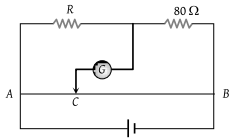(1) 2 Ω

(2) 8 Ω

(3) 20 Ω

(4) 40 Ω

Concept Questions :-

Meter bridge and potentiometer
High Yielding Test Series + Question Bank - NEET 2020

Difficulty Level:

The circuit shown here is used to compare the e.m.f. of two cells ${E}_{1}$ and ${E}_{2}\left({E}_{1}>{E}_{2}\right)$. The null point is at C when the galvanometer is connected to E1. When the galvanometer is connected to E2, the null point will be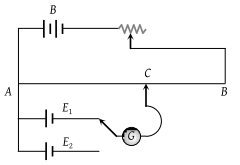(1) To the left of C

(2) To the right of C

(3) At C itself

(4) Nowhere on AB

Concept Questions :-

Meter bridge and potentiometer
High Yielding Test Series + Question Bank - NEET 2020

Difficulty Level:

In the Wheatstone's bridge (shown in figure) X = Y and A > B. The direction of the current between ab will be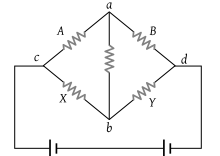(1) From a to b

(2) From b to a

(3) From b to a through c

(4) From a to b through c

Concept Questions :-

Wheatstone bridge
High Yielding Test Series + Question Bank - NEET 2020

Difficulty Level:

A resistance of 4 Ω and a wire of length 5 metres and resistance 5 Ω are joined in series and connected to a cell of e.m.f. 10 V and internal resistance 1 Ω. A parallel combination of two identical cells is balanced across 300 cm of the wire. The e.m.f. E of each cell is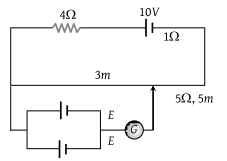(1) 1.5 V

(2) 3.0 V

(3) 0.67 V

(4) 1.33 V

Concept Questions :-

Meter bridge and potentiometer
High Yielding Test Series + Question Bank - NEET 2020

Difficulty Level:

Constantan wire is used in making standard resistances because of its :

(1) Specific resistance is low

(2) Density is high

(3) Temperature coefficient of resistance is negligible

(4) Melting point is high

Concept Questions :-

Derivation of Ohm's law
High Yielding Test Series + Question Bank - NEET 2020

Difficulty Level:

A potentiometer consists of a wire of length 4 m and resistance 10 Ω. It is connected to a cell of e.m.f. 2 V. The potential difference per unit length of the wire will be :

(1) 0.5 V/m

(2) 2 V/m

(3) 5 V/m

(4) 10 V/m

Concept Questions :-

Meter bridge and potentiometer
High Yielding Test Series + Question Bank - NEET 2020

Difficulty Level:

In the circuit shown $P\ne R$, the reading of the galvanometer is same with switch S open or closed. Then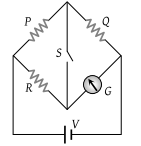(1) ${I}_{R}={I}_{G}$

(2) ${I}_{P}={I}_{G}$

(3) ${I}_{Q}={I}_{G}$

(4) ${I}_{Q}={I}_{R}$

Concept Questions :-

Wheatstone bridge
High Yielding Test Series + Question Bank - NEET 2020

Difficulty Level:

In the following Wheatstone bridge $P/Q=R/S$. If key K is closed, then the galvanometer will show deflection :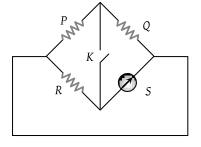(1) In left side

(2) In right side

(3) No deflection

(4) In either side

Concept Questions :-

Wheatstone bridge
High Yielding Test Series + Question Bank - NEET 2020

Difficulty Level:

In a potentiometer experiment, the galvanometer shows no deflection when a cell is connected across 60 cm of the potentiometer wire. If the cell is shunted by a resistance of 6 Ω, the balance is obtained across 50 cm of the wire. The internal resistance of the cell is

(1) 0.5 Ω

(2) 0.6 Ω

(3) 1.2 Ω

(4) 1.5 Ω

Concept Questions :-

Meter bridge and potentiometer